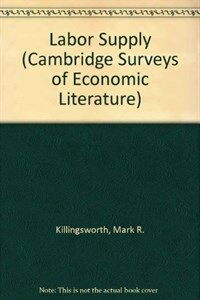> 상세정보

# 상세정보## Labor supply (28회 대출)

자료유형
단행본
개인저자
Killingsworth, Mark R., 1946-
서명 / 저자사항
Labor supply / Mark R. Killingsworth.
발행사항
Cambridge [Cambridgeshire] ;   New York :   Cambridge University Press,   1983.
형태사항
xvi, 493 p. : ill. ; 22 cm.
총서사항
Cambridge surveys of economic literature
ISBN
0521233267 0521299160 (pbk.)
일반주기
Includes indexes.
서지주기
Bibliography: p. 446-478.
일반주제명
Labor supply.
비통제주제어
Labour market, Econometric models,,
 000 00908pamuuu200301 a 4500 001 000000118254 005 19950929053001.0 008 820729s1983 enka b 001 0 eng 010 ▼a 82014598 015 ▼a GB84-32779 019 ▼a 12461123 020 ▼a 0521233267 020 ▼a 0521299160 (pbk.) 035 ▼a ocm08689588 040 ▼a DLC ▼c DLC ▼d UKM 049 ▼a ACCL ▼l 474349209 ▼x A 050 0 ▼a HD5706 ▼b .K57 1983 082 0 ▼a 331.12 ▼2 19 090 ▼a 331.12 ▼b K48L 100 1 ▼a Killingsworth, Mark R., ▼d 1946- 245 1 0 ▼a Labor supply / ▼c Mark R. Killingsworth. 260 ▼a Cambridge [Cambridgeshire] ; ▼a New York : ▼b Cambridge University Press, ▼c 1983. 300 ▼a xvi, 493 p. : ▼b ill. ; ▼c 22 cm. 440 0 ▼a Cambridge surveys of economic literature 500 ▼a Includes indexes. 504 ▼a Bibliography: p. 446-478. 650 0 ▼a Labor supply. 653 ▼a Labour market ▼a Econometric models

### 소장정보

No. 소장처 청구기호 등록번호 도서상태 반납예정일 예약 서비스
No. 1 소장처 청구기호 331.12 K48L 등록번호 474349209 도서상태 대출가능 반납예정일 예약 서비스

### 컨텐츠정보

#### 목차

```
CONTENTS
List of tables and figures = ⅹ
Preface = xiii
1 The simple static model of labor supply = 1
2 Extensions of the simple short-run model of labor supply = 29
2.1 Labor supply of family members = 29
2.2 Labor supply and the allocation of time = 38
2.3 Dimensions of labor supply : hours, weeks, years, lifetimes = 43
2.4 The discontinuous budget line : nontangencies, rationing, unemployment, and "disequilibrium" = 45
3 Empirical studies of static labor supply models : introduction, with a summary of "first-generation" results = 67
3.1 Econometric specification = 68
3.2 Sample selection = 78
3.3 Problems of measurement = 87
3.4 Results of first-generation studies = 100
3.5 First-generation studies : summary and conclusions = 127
4 Second-generation studies of static labor supply models : methodology and empirical results = 130
4.1 The static labor supply model revisited = 132
4.2 Second-generation estimation techniques : general principles = 135
4.3 Applications to the simple labor supply model = 150
4.4 Applications to discontinuous labor supply schedules = 157
4.5 Applications to labor supply models with taxes = 168
4.6 Results of second-generation labor supply studies =179
4.7 Second-generation studies : summary and conclusions = 205
5  Dynamic labor supply models = 207
5.1 Dynamic labor supply models : an overview = 209
5.2 Dynamic labor supply models with exogenous wages : theory = 214
5.3 Risk and uncertainty = 239
5.4 Dynamic labor supply models with exogenous wages : empirical analysis = 263
5.5 Human capital : labor supply with endogenous wages = 297
5.6 Dynamic labor supply models with endogenous wages : theory = 310
5.7 Dynamic labor supply models with endogenous wages : empirical analysis = 316
6 Labor supply, taxes, and transfers = 331
6.1 Labor supply effects of taxes and transfers : analytical framework : 332
6.2 Income taxes and labor supply =352
6.3 Transfer payments and labor supply : introduction = 360
6.4 Transfer payments and labor supply : empirical analysis = 375
6.5 Negative income tax experimental results = 392
6.6 Social security and labor supply = 409
6.7 Other transfer programs and labor supply = 420
7 Conclusion = 431
7.1 What we do(and don't) know about labor supply = 432
7.2 Where do we go from here? = 441
Bibliography = 446
Name index = 479
Subject index = 000

```

### 관련분야 신착자료

홍현균 (2020)

정순기 (2020)

#### 불쉿 잡 : 왜 무의미한 일자리가 계속 유지되는가?

Graeber, David (2021)

홍명교 (2021)

장재호 (2020)

#### 2020년도 연구분야별 주요 연구결과 요약집

국민연금공단. 국민연금연구원 (2021)

남보라 (2021)

유경현 (2021)

김명수 (2021)

#### International & comparative employment relations : global crises and institutional responses / 7th ed

Bamber, Greg (2021)

백완기 (2021)

이은혜 (2021)

황정욱 (2021)

권도형 (2021)

조은영 (2021)

최영민 (2021)

황정욱 (2021)

신경혜 (2021)